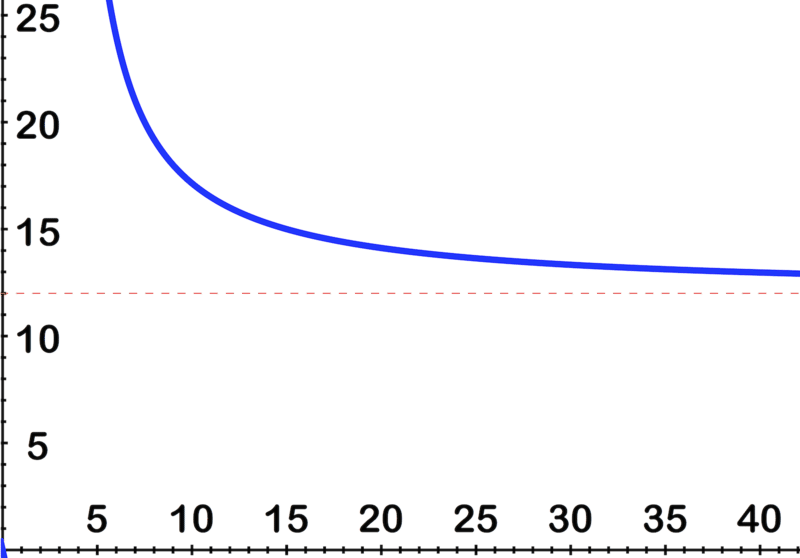# 8.1.3: Infinite Limits

$$\newcommand{\vecs}{\overset { \rightharpoonup} {\mathbf{#1}} }$$ $$\newcommand{\vecd}{\overset{-\!-\!\rightharpoonup}{\vphantom{a}\smash {#1}}}$$$$\newcommand{\id}{\mathrm{id}}$$ $$\newcommand{\Span}{\mathrm{span}}$$ $$\newcommand{\kernel}{\mathrm{null}\,}$$ $$\newcommand{\range}{\mathrm{range}\,}$$ $$\newcommand{\RealPart}{\mathrm{Re}}$$ $$\newcommand{\ImaginaryPart}{\mathrm{Im}}$$ $$\newcommand{\Argument}{\mathrm{Arg}}$$ $$\newcommand{\norm}{\| #1 \|}$$ $$\newcommand{\inner}{\langle #1, #2 \rangle}$$ $$\newcommand{\Span}{\mathrm{span}}$$ $$\newcommand{\id}{\mathrm{id}}$$ $$\newcommand{\Span}{\mathrm{span}}$$ $$\newcommand{\kernel}{\mathrm{null}\,}$$ $$\newcommand{\range}{\mathrm{range}\,}$$ $$\newcommand{\RealPart}{\mathrm{Re}}$$ $$\newcommand{\ImaginaryPart}{\mathrm{Im}}$$ $$\newcommand{\Argument}{\mathrm{Arg}}$$ $$\newcommand{\norm}{\| #1 \|}$$ $$\newcommand{\inner}{\langle #1, #2 \rangle}$$ $$\newcommand{\Span}{\mathrm{span}}$$$$\newcommand{\AA}{\unicode[.8,0]{x212B}}$$

## Infinite Limits

Geeks-R-Us sells titanium mechanical pencils to computer algorithm designers. In an effort to attract more business, they decide to run a rather unusual promotion:

"SALE!! The more you buy, the more you save! Pencils are now $$\ \ \frac{12 x}{x-3}$$ per dozen!"

If the trillionaire, Spug Dense, comes in and says he wants to buy as many pencils as Geeks-R-Us can turn out, what will the cost of the pencils approach as the order gets bigger and bigger?

## Infinite Limits

Sometimes, a function may not be defined at a particular number, but as values are input closer and closer to the undefined number, a limit on the output may not exist. For example, for the function f(x) = 1/x (shown in the figures below), as x values are taken closer and closer to 0 from the right, the function increases indefinitely. Also, as x values are taken closer and closer to 0 from the left, the function decreases indefinitely.We describe these limiting behaviors by writing

$$\ \begin{array}{l} \lim _{x \rightarrow 0^{+}} \frac{1}{x}=+\infty \\ \lim _{x \rightarrow 0^{-}} \frac{1}{x}=-\infty \end{array}$$

Sometimes we want to know the behavior of $$\ f(x)$$ as x increases or decreases without bound. In this case we are interested in the end behavior of the function, a concept you have likely explored before. For example, what is the value of $$\ f(x)=1 / x$$ as $$\ x$$ increases or decreases without bound? That is,

$$\ \begin{array}{l} \lim _{x \rightarrow+\infty} \frac{1}{x}=? \\ \lim _{x \rightarrow-\infty} \frac{1}{x}=? \end{array}$$

As you can see from the graphs (shown below), as $$\ x$$ decreases without bound, the values of $$\ f(x) = 1/x$$ are negative and get closer and closer to 0. On the other hand, as $$\ x$$ increases without bound, the values of $$\ f(x) = 1/x$$ are positive and still get closer and closer to 0.That is,

$$\ \begin{array}{l} \lim _{x \rightarrow+\infty} \frac{1}{x}=0 \\ \lim _{x \rightarrow-\infty} \frac{1}{x}=0 \end{array}$$

## Examples

###### Example 1

Solution

As Spug buys more and more pencils, the cost of each dozen will drop quickly at first, and level out after a while, approaching \$12 per dozen.

You can see the effect on the graph here:###### Example 2

Evaluate the limit by making a graph: $$\ \lim _{x \rightarrow 3^{+}} \frac{x+6}{x-3}$$

Solution

By looking at the graph:We can see that as x gets closer and closer to 3 from the positive side, the output increases right out the top of the image, on its way to ∞

###### Example 3

Evaluate the limit: $$\ \lim _{x \rightarrow \infty} \frac{11 x^{3}-14 x^{2}+8 x+16}{9 x-3}$$.

Solution

To evaluate polynomial function limits, a little bit of intuition helps. Let's think this one through.

First, note that since we are looking at what happens as $$\ x \rightarrow \infty$$ most of the interesting stuff will happen as $$\ x$$ gets really big.

On the top part of the fraction, as x gets truly massive, the $$\ 11 x^{3}$$ part will get bigger much faster than either of the other terms. In fact, it increases so much faster than the other terms completely cease to matter at all once x gets really monstrous. That means that the important part of the top of the fraction is just the $$\ 11 x^{3}$$.

On the bottom, a similar situation develops. As x gets really, really big, the -3 matters less and less. So the bottom may as well be just $$\ 9x$$.

That gives us $$\ \frac{11 x^{3}}{9 x}$$ which reduces to $$\ \frac{11 x^{2}}{9}$$

Now we can more easily see what happens at the "ends." As x gets bigger and bigger, the numerator continues to get bigger faster than the denominator, so the overall output also increases.

$$\ \therefore \lim _{x \rightarrow+\infty} \frac{11 x^{3}-14 x^{2}+8 x+16}{9 x-3} \text { is }+\infty$$

###### Example 4

Evaluate $$\ \lim _{x \rightarrow 0} \frac{x+2}{x+3}$$

Solution

This one is easier than it looks! As x-->0, leaving just the fraction: 2/3

###### Example 5

Make a graph to evaluate the limit $$\ \lim _{x \rightarrow \infty} \frac{1}{\sqrt{x}}$$ and $$\ \lim _{x \rightarrow 0^{+}} \frac{1}{\sqrt{x}}$$.

Solution

By looking at the image, we see that as x gets huge, so does $$\ \sqrt{x}$$ which means that 1 is being divided by an ever-larger number, and the result is getting smaller and smaller.

$$\ \lim _{x \rightarrow \infty} \frac{1}{\sqrt{x}}=0$$On the same image, we can see that as $$\ x$$ gets closer and closer to zero, so does $$\ \sqrt{x}$$ which means that 1 is being divided by an ever smaller number, and the result gets bigger and bigger.

$$\ \lim _{x \rightarrow 0^{+}} \frac{1}{\sqrt{x}} \text { is }+\infty$$###### Example 6

Graph and evaluate the limit: $$\ \lim _{x \rightarrow 2^{+}} \frac{1}{x-2}$$.

Solution

By looking at the image, we can see that as x gets closer and closer to 2 from the positive direction, 1 gets divided by smaller and smaller numbers, so the result gets larger and larger.$$\ \lim _{x \rightarrow 2^{+}} \frac{1}{x-2} \text { is }+\infty$$

## Review

Evaluate the limits:

1. $$\ \lim _{x \rightarrow 3^{-}} \frac{1}{x-3}$$
2. $$\ \lim _{x \rightarrow-4^{+}} \frac{1}{x+4}$$
3. $$\ \lim _{x \rightarrow-\left(\frac{8}{3}\right)^{+}} \frac{1}{3 x+8}$$
4. $$\ \lim _{x \rightarrow-5^{+}} \frac{\left(x^{2}+11 x+30\right)}{x+5}$$
5. $$\ \lim _{x \rightarrow-\infty} \frac{\left(x^{2}+11 x+30\right)}{x+5}$$
6. $$\ \lim _{x \rightarrow \infty} \frac{-11 x^{3}+20 x^{2}+15 x-17}{-9 x^{3}+5 x^{2}-x-17}$$
7. $$\ \lim _{x \rightarrow \infty} 13$$
8. $$\ \lim _{x \rightarrow \infty} \frac{-2 x+18}{17 x-3}$$
9. $$\ \lim _{x \rightarrow \infty} 15$$
10. $$\ \lim _{x \rightarrow \infty}-5 x^{2}+5 x+14$$
11. $$\ \lim _{x \rightarrow \infty} 7 x+12$$
12. $$\ \lim _{x \rightarrow \infty}-3 x+13$$
13. $$\ \lim _{x \rightarrow \infty} \frac{13 x-8}{19 x^{3}-11 x^{2}+x+4}$$
14. $$\ \lim _{x \rightarrow \infty}-17 x+14$$
15. $$\ \lim _{x \rightarrow \infty}-7 x^{2}-2 x-13$$